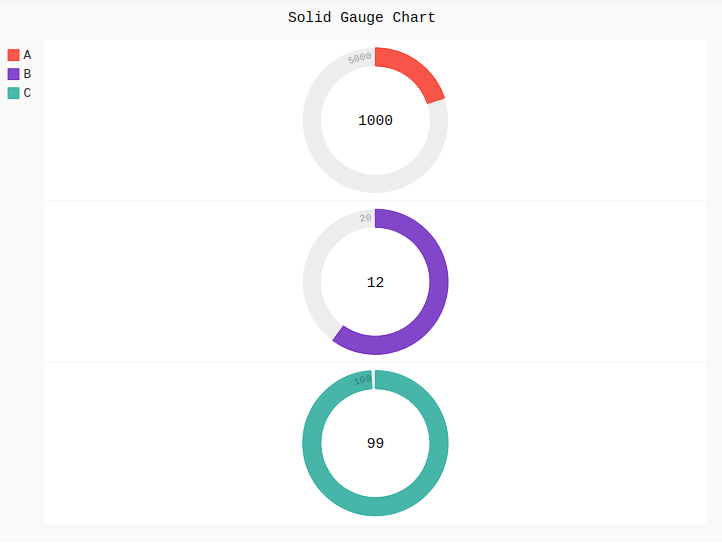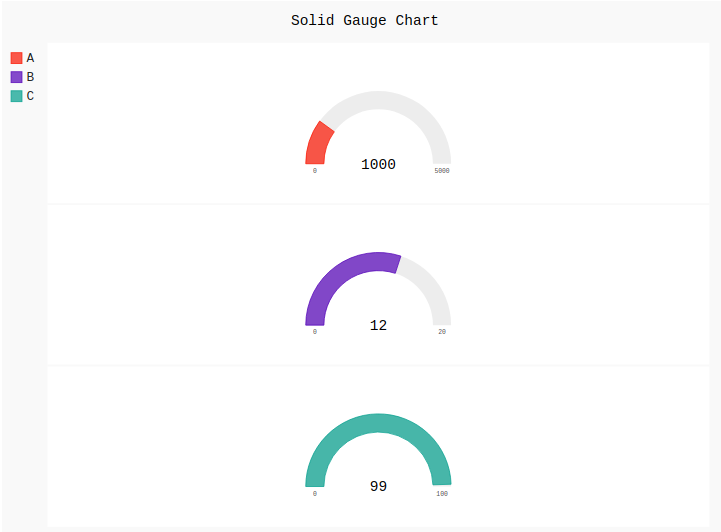# Solid Gauge Chart in Pygal

Pygal is a Python module that is mainly used to build SVG (Scalar Vector Graphics) graphs and charts. SVG is a vector-based graphics in the XML format that can be edited in any editor. Pygal can create graphs with minimal lines of code that can be easy to understand and write.

## Solid Gauge Chart

Gauges are the popular charts which help for dashboards, they anticipate a number in a range at a glance. It can use colored bands, hands, and combinations to display multiple values and their relation to a numeric scale.

Syntax:

```gauge = pygal.SolidGauge()
```

Example 1:

## Python3

 `# importing pygal ` `import` `pygal ` ` `  `# creating the chart object ` `Solid_Gauge ``=` `pygal.SolidGauge(inner_radius ``=` `0.75``) ` ` `  `# naming the title ` `Solid_Gauge.title ``=` `'Solid Gauge Chart'`      ` `  `# Random data ` `Solid_Gauge.add(``'A'``, [{``'value'``: ``1000``, ``'max_value'``: ``5000``}]) ` `Solid_Gauge.add(``'B'``, [{``'value'``: ``12``, ``'max_value'``: ``20``}]) ` `Solid_Gauge.add(``'C'``, [{``'value'``: ``99``, ``'max_value'``: ``100``}]) ` ` `  `Solid_Gauge`

Output:Example 2:

## Python3

 `# importing pygal ` `import` `pygal ` ` `  `# creating the chart object ` `Solid_Gauge ``=` `pygal.SolidGauge(inner_radius ``=` `0.75``,  ` `                               ``half_pie ``=` `True``) ` ` `  `# naming the title ` `Solid_Gauge.title ``=` `'Solid Gauge Chart'`      ` `  `# Random data ` `Solid_Gauge.add(``'A'``, [{``'value'``: ``1000``, ``'max_value'``: ``5000``}]) ` `Solid_Gauge.add(``'B'``, [{``'value'``: ``12``, ``'max_value'``: ``20``}]) ` `Solid_Gauge.add(``'C'``, [{``'value'``: ``99``, ``'max_value'``: ``100``}]) ` ` `  `Solid_Gauge`

Output:Whether you're preparing for your first job interview or aiming to upskill in this ever-evolving tech landscape, GeeksforGeeks Courses are your key to success. We provide top-quality content at affordable prices, all geared towards accelerating your growth in a time-bound manner. Join the millions we've already empowered, and we're here to do the same for you. Don't miss out - check it out now!